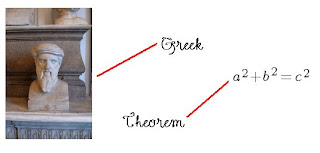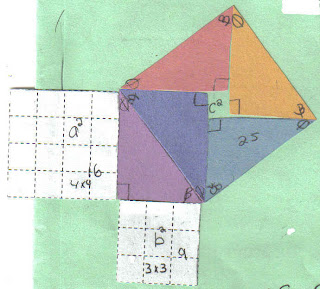### Pythagoras

Monday, February 23, 2009
Okay so Mr. Harbeck asked us to make a post relating the words and the pictures to all make sense in the end. Here is my post.

I'm going to start with the Right Triangle.So as you can see it the picture above it labels are 3 out of 5 vocabulary words that were need to use and one picture. I'll explain them a bit better.

Hypotenuse- The Hypotenuse is the longest side in every right triangle. It is always located across from the right angle and would be label 'c' in the equation(later in the post).

Legs- They make up the 90° angle and are always labeled 'a' and 'b'(Later).

R.A.T- R.A.T means Right Angle Triangle.

Next that last two words explained and two more pictures.Okay so the word Greek is related to the whole thing because that statue is of a Greek mathematician, Pythagoras. Pythagoras has a theorem 'a² + b² = c²' named Pythagoras' Theorem.This man proved that he could turn four right angles into a square and vise versa.

So this is the idea.This is the Theorem. Now the idea is to make it into a whole bunch of triangles like so:Here are a couple of videos that Jordan and I made in class.
The first movie explains how a² + b² = c² and the second on explains how to find out what b² is.

Movie One:

Movie Two: